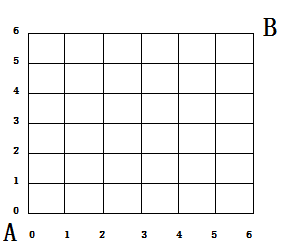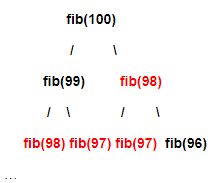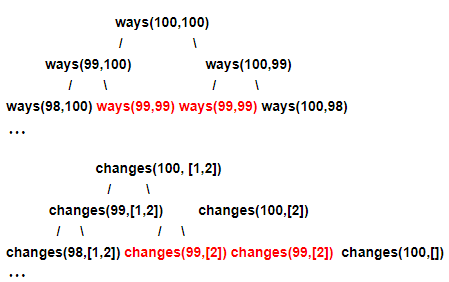版权申明：本文为博主窗户(Colin Cai)原创，欢迎转帖。如要转贴，必须注明原文网址

http://www.cnblogs.com/Colin-Cai/p/13499260.html

作者：窗户

QQ/微信：6679072

E-mail：6679072@qq.com

本系列文章是想思考思考递归的编译优化问题，目标在于希望如何从编译、解释层次将树递归进行优化，从而避免过低效率运行。本章来讲讲树递归的问题。

几个递归问题

先来看这样一个知名数列Fibnacci数列，定义如下

$fib_{n} = \left\{\begin{matrix} 1， & n = 1,2\\ fib_{n-1}+fib_{n-2},& n>3 \end{matrix}\right.$

获得数列第n项用程序写如下，以下可以看成是伪码，只是用Python写出来，其实用什么语言写出来对于本文章所述说内容来说没太大关系：

def fib(n):
if n < 3:
return 1
return fib(n-1) + fib(n-2)

再来看另外一个问题，如下图这样的方格，从A走到B，每次往右走一步或每次往上走一步，问有多少走法。稍微加分析我们就可以知道，对于从(0,0)到坐标(x, y)的走法数量：

(1)如果在两条坐标轴上，也就是x=0或者y=0，那么只有一种走法

(2)除此之外，任何一种走法走到(x,y)之前的上一步，则要么是走到了(x-1,y)，要么是走到了(x,y-1)。从而，这种情况下，走到(x,y)的走法数量应该是走到(x-1,y)的走法数量和走到(x,y-1)的走法数量之和。

用程序来表达，应该是

def ways(x, y):
if x==0 or y==0:
return 1
else:
return ways(x-1, y) + ways(x, y-1)

再看一个问题，可以称之为零钱问题。假如有1分、2分、5分、10分、20分、50分、100分、200分、500分、1000分这几种面值各不相同的货币，组成1000分一共有多少种方法。

这个问题一眼看上去可能会觉得毫无头绪，但依然存在树递归的方法。我们把原问题看成是changes(total, notes)，total为希望组成的钱的数量，notes是各种货币面值组成的list，其递归思路如下：

(1)如果total=0，则组成方法当然只有一种。

(2)如果total<0，则组成方法一种都没有。

(3)如果total≥0且notes里面一种货币都没有，则组成方法也是一种都没有。

(4)其他情况下，从notes中拿出一种货币，那么所有的组成方法包含两类，一类包含刚才这种货币，一类不包含刚才这种货币，两类情况交集为空。

用程序来实现这个递归如下：

def changes(total, notes):
if total == 0:
return 1
if total < 0:
return 0
if len(notes) == 0:
return 0
return changes(total-notes, notes) \
+ changes(total, notes[1:])

原问题则可以靠changes(1000, [1,2,5,10,20,50,100,200,500,1000])这样去求值了，其中第二个list的顺序并不重要。

递归的效率

实际上，上述的三个Python代码执行以下三个函数调用

fib(100)

ways(100, 100)

changes(1000, [1,2,5,10,20,50,100,200,500,1000])

就可以看出问题，因为这三个函数调用似乎结束不了，最后一个可能需要修改一下栈大小。

一个纯计算的函数的执行卡死，可能是执行运算量过大了。

我们这里只考虑一下fib函数，其他两个类比。

实际上，我们单修改一下，添加一个计数cnt来记录fib被调用的次数，用来估算时间复杂度，

cnt = 0
def fib(n):
global cnt
cnt += 1
if n < 3:
return 1
return fib(n-1) + fib(n-2)

我们计算一下fib(10)，得到55

打印cnt，得到109。

实际上，cnt显然是以下这样一个数列

$cnt_{n} = \left\{\begin{matrix} 1， & n = 1,2\\ cnt_{n-1}+cnt_{n-2}+1,& n>3 \end{matrix}\right.$

很容易用数学归纳法证明

$cnt_{n}=fib_{n}*2-1$

而fib是指数级的数列，所以这个树递归的计算fib(n)也是n的指数级的计算量，这个当然就可怕了。其他两个也一样是类似的计算量，从而短时间计算不出来是很正常的。

递归为什么如此慢

为什么这些递归会如此的慢呢？这是一个值得思考的问题，也是提升其效率的入手点。

我们还是从Fibnacci数列开始研究起，我们刚才知道了函数会被调用很多次，于是我们就想，每次调用函数的时候，参数都是什么。修改一下:

def fib(n):
print('Call fib, arg:', n)
if n < 3:
return 1
return fib(n-1) + fib(n-2)

我们执行一下fib(6)，得到下面的打印结果：

Call fib, arg: 6
Call fib, arg: 5
Call fib, arg: 4
Call fib, arg: 3
Call fib, arg: 2
Call fib, arg: 1
Call fib, arg: 2
Call fib, arg: 3
Call fib, arg: 2
Call fib, arg: 1
Call fib, arg: 4
Call fib, arg: 3
Call fib, arg: 2
Call fib, arg: 1
Call fib, arg: 2

我们观察可以发现，一样参数的fib调用总是频繁发生，我们排除fib(1)、fib(2)这种可以直接得到结果的调用，fib(4)被调用了2次，fib(3)被调用了3次。然而，显然这个函数不包含任何的副作用，也就是函数本身的运算不会影响任何全局变量，所使用的运算部件也不带有任何的随机成分等。那么也就是，这样的函数是数学意义上的函数，所谓“纯函数”，从而相同的参数会计算出相同的值。

比如fib(100)，以下是计算中的递归依赖红色的部分都是重复计算，大量的重复计算导致了计算效率过低，同样的事情也发生在ways和changes两个函数上。如何避免重复

我们可以在黑板上依次根据规则一项项的写出Fibnacci数列各项，

1 1 2 3 5 8 13 21 34 55 89 144 ...

可以预计，一个小时差不多可以写出第100项，于是人比计算机快？

其实，还是在于人没有重复计算，当然人在这里采用了一个更好的迭代算法也是一个原因。

于是，我们可以想到，之前我们已经分析这些函数都是数学意义下的函数，如果建立一个cache，记录下函数得到的值，每次计算函数，当可能出现递归的时候，都先去查一下cache，如果cache中有，则取出返回，如果没有则递归计算。

fib函数可以按照以上想法改写为这样，

cache = {}
def fib(n):
if n < 3:
return 1
if n in cache:
return cache[n]
else:
r = fib(n-1) + fib(n-2)
cache[n] = r
return r

以此算法来运算fib(100)发现可以瞬间得到354224848179261915075

依然以之前的方法记录一下函数调用次数，

cache = {}
cnt = 0
def fib(n):
global cnt
cnt += 1
if n < 3:
return 1
if n in cache:
return cache[n]
else:
r = fib(n-1) + fib(n-2)
cache[n] = r
return r

发现计算fib(100)之后，cnt只记录到197，显然cache避免了大量重复计算，从而很快。

编译器判断一个函数是数学函数从而没有副作用其实并不难，只需要满足如下：

(1)函数和全局变量不产生直接交互

(2)函数如果有使用到其他函数，这些外部函数也是数学函数。

(3)函数如果用到操作，所使用的操作不产生副作用。

实际上，可以把操作也看成函数，从而只有上述1、2两条。然后这个cache也是一个k/v系统，此种优化可以在编译器中直接做到。

甚至还可以考虑引入多任务，不过这是个比较麻烦的问题。另外，这种优化并不一定总是可以带来更高的效率，如果没有上述的大量复重复计算，这样的优化反而会加大数据复杂度开销，也加长运算时间。

我这里用阶乘举个例：

def factorial(n):
if n == 1:
return 1
return n * factorial(n-1)

以上递归并没有重复计算，添加cache如下：

cache = {}
def factorial(n):
if n == 1:
return 1
if n in cache:
print('Cache Hit')
return cache[n]
r = n * factorial(n-1)
cache[n] = r
return r

因为没有重复计算，所以上面的Cache Hit永远不可能打印。

试图追求更高的效率

前面提到可以在黑板上一项一项写出Fibnacci数列，用到的方法是迭代，用Python使用递归形式来描述迭代如下：

def fib(n):
def fib_iter(n, m, first, second):
if n == m:
return second
return fib_iter(n, m+1, second, second+first)
if n < 3:
return 1
return fib_iter(n, 2, 1, 1)

而用循环来描述迭代如下：

def fib(n):
if n < 3:
return 1
first = 1
second = 1
for i in range(3, n+1):
first, second = second, second+first
return second

虽说对于Fibnacci数列求第n项有更好(时间复杂度更低)的算法，但是如果编译器可以自动产生以上算法，已经是可以满意了。

我们思考用递归计算Fibnacci数列中的一项fib(n)

以下符号，->左边代表目标，->右边::左边代表依赖值，::右边代表函数，

fib(n)->fib(n-1) ,fib(n-2)::λx y·x+y

而所依赖的两个值分别是如下依赖，

fib(n-1)->fib(n-2),fib(n-3)::λx y·x+y

fib(n-2)->fib(n-3),fib(n-4)::λx y·x+y

从而

fib(n-1),fib(n-2)->fib(n-3),fib(n-4)::λx y·x+y+x, λx y·x+y

于是我们反复来就可以有以下的依赖

fib(n)->fib(n-1) ,fib(n-2)::λx y·x+y

fib(n-1),fib(n-2)->fib(n-3),fib(n-4)::λx y·x+y+x, λx y·x+y

fib(n-3),fib(n-4)->fib(n-5),fib(n-6)::λx y·x+y+x, λx y·x+y

...

于是我们的依赖

fib(n)->fib(n-1),fib(n-2)->fib(n-3),fib(n-4)->fib(n-5,n-6)...

反过来

f(1),f(2)=>f(3),f(4)=>f(5),f(6)....f(n)

于是我们就有了一个O(1)空间的迭代，然而问题在于，我们怎么知道反过来可以从f(1),f(2)开始推呢？

而考虑第二个问题ways递归，问题则变得麻烦了许多。

ways(a,b)->ways(a-1,b),ways(a,b-1)::λx y·x+y

而

ways(a-1,b)->ways(a-2,b),ways(a-1,b-1)::λx y·x+y

ways(a,b-1)->ways(a-1,b-1),ways(a,b-2)::λx y·x+y

从而

ways(a-1,b),ways(a,b-1)->ways(a-2,b),ways(a-1,b-1),ways(a,b-2)::λx y z·x+y,λx y z·y+z

....

于是我们通过观察，可以反过来这样去推：

f(1,1)=>f(2,1),f(1,2)=>f(3,1),f(2,2),f(3,3)=>....

其中省略所有的递归边界条件，比如

f(2,1) = f(1,1)+f(2,0) = f(1,1)+1

于是，这几乎成了一个人脑才能理解的问题，很难有固定的算法可以将树递归转换为迭代，不过得到一种人脑似乎可以通过树递归寻找迭代的方法，也算不错。

当然，编译器大多数优化方法还是使用粒度更细的模板式寻找和替换，没有通式的优化，可以采用模板式的匹配，替换。

posted on 2020-08-31 22:30  窗户  阅读(676)  评论(0编辑  收藏  举报